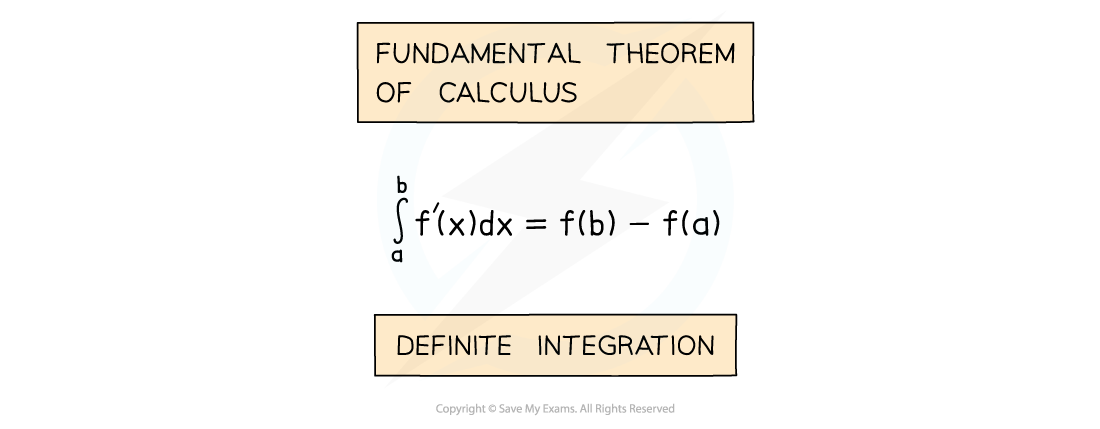# AQA A Level Maths: Pure复习笔记8.1.3 Definite Integration

### Definite Integration

#### What is definite integration?

• Definite Integration occurs in an alternative version of the Fundamental Theorem of Calculus
• This version of the Theorem is the one referred to by most AS/A level textbooks/websites• a and b are called limits
• a is the lower limit
• b is the upper limit
• f’(x) is the derivative of f(x)

#### What happened to c, the constant of integration?•  “+c” would appear in both f(a) and f(b)
• Since we then calculate f(b) – f(a) they cancel each other out
• There would be a “+c” from f(b) and a –“+c” from f(a)
• So “+c” is not included with definite integration

#### How do I find a definite integral?

• STEP 1: If not given a name, call the integral
• This saves you having to rewrite the whole integral every time!
• STEP 2:  If necessary rewrite the integral into a more easily integrable form
• Not all functions can be integrated directly
• STEP 3:  Integrate without applying the limits
• Notation: use square brackets [ ] with limits placed after the end bracket
• STEP 4:  Substitute the limits into the function and calculate the answer#### Using a calculator

• Advanced scientific calculators can work out the values of definite integrals
• The button will look similar to:•  (Note how the calculator did not return the exact value [Image_Link]data:;base64,